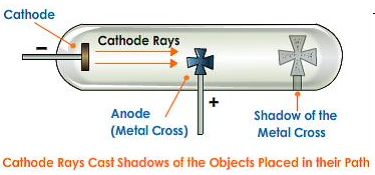### Atoms and Molecules - Test Papers

CBSE Test Paper 01

Chapter 03 Atoms and Molecules

1. A sample of CaCO3 contains  ions of Ca+2 and CO3-2. The mass of the sample is: (1)

1. 200 g
2. 50 g
3. 100 g
4. 5 g
2. SO3 is (1)

1. acidic
2. amphoteric
3. basic
4. neutral
3. When dilute sulphuric acid is added to zinc granules, we observe that: (1)

1. the container remains cool
2. the reaction mixture turns yellow
3. bubbles start coming from the surface of the zinc granules
4. a precipitate is formed
4. The number of molecules in CuSO4.5H2O bonded by H-bond is (1)

1. 5
2. 3
3. 2
4. 1
5. The maximum number of electrons in L shell is: (1)

1. 38
2. 28
3. 18
4. 8
6. An element X has valency 3 while the element Y has valency 2. Write the formula of the compound formed by X and Y. (1)

1. X2Y2
2. X3Y3
3. XY
4. X2Y3
7. Define atomic mass unit (1)

8. How many atoms are present in a: (1)

1. H2S molecule and
2. $P{O}_{4}^{3-}$ ion?
9. Name the Indian philosopher who proposed the theory of matter. (1)

10. Find the ratio by mass of the elements present in the molecule of hydrogen sulphide (H2S). Given that, atomic mass S = 32, H = 1. (1)

11. What is the difference between Hand 2H? (3)

12. Calculate the number of moles in the following : (3)

1. 28g He
2. 46g of Na.
3. 60 g of Ca.

Given the gram atomic mass of

1. He = 4 g
2. Na = 23 g
3. Ca = 40g.
13. Calculate the molar mass of ethyl alcohol (C2H5OH). (3)

14. Write the chemical formulae of the following. (5)

1. Magnesium chloride
2. Calcium oxide
3. Copper(II) nitrate
4. Aluminium chloride
5. Calcium carbonate.
15. Write an experiment to show that cathode rays travel in straight line? (5)

CBSE Test Paper 01
Chapter 03 Atoms and Molecules

1. 50 g
Explanation: The mass of one mole of CaCO3 is equal to 100 g.  ions are equivalent to one mole. Therefore, mass of $3.01×{10}^{23}$ ions will be equivalent to (100 / 2) g or 50 g.
1. acidic
Explanation: Molecules whose Lewis structures indicate an atom to have an octet as a result of the formation of one or more multiple bonds will often function as Lewis acids. Examples are CO2, SO3, SO2.
SO3 is acidic in nature as it is non-metallic oxide.
1. bubbles start coming from the surface of the zinc granules
Explanation: Zinc reacts with dil. H2SO4 to form H2 gas and we observe bubbles start coming from the surface of the zinc granules.
Zn(s) + H2SO4 →ZnSO4(aq) + H2(g)
This reaction is exothermic and hence heat is evolved in the reaction.
1. 1
Explanation: One molecule of water is linked by H-bond because only one molecule of water is present outside the coordination sphere.
1. 8
Explanation: L shell can accomodate a maximum of 8 electrons.
1. X2Y3
Explanation: The formula of an ionic compound is formed by interchanging the valencies of the constituent atoms. The formula of the compound having atoms of X and Y is X2Y3. The valency (2) of Y forms the subscript of X and the valency (3) of X forms the subscript of Y.
1. Atomic mass unit may be defined as:
The mass of one twelfth (1/12) of the mass of one atom of carbon taken as 12u. It is represented as 1u.

1. 2 atoms of hydrogen + 1 atom of sulphur = 3 atoms. Hence 3 atoms are present in an H2S molecule.
2. 1 atom of phosphorus + 4 atoms of oxygen = 5 atoms. Hence 5 atoms are present in an ion.$P{O}_{4}^{3-}$
2. Kanad proposed the theory of matter around 600 BC.

3. atomic mass of sulphur S = 32 u
atomic mass of hydrogen H = 1u
the ratio by mass of the elements present in the molecule of hydrogen sulphide (H2S) = mass of hydrogen atom present in the compound : the mass of the sulphur atom present compound
= 2$×$1 : 32 = 2 : 32 = 1 : 16

4. Hrepresents one molecule of H(hydrogen gas) whereas 2H represents two separate atoms of hydrogen.

1. 28 g of He
The no. of moles
= $\frac{m}{M}$$\frac{\left(28\mathrm{g}\right)}{\left(4\mathrm{g}\right)}$= 7 mol
2. 46 g of Na
The no. of moles
= $\frac{m}{M}$ = $\frac{\left(46g\right)}{\left(23g\right)}$ = 2 mol
3. 60 g of Ca
The no. of moles
= $\frac{m}{M}$ = $\frac{\left(60\mathrm{g}\right)}{\left(40\mathrm{g}\right)}$ = 1.5 mol
5. molar mass of C2H5OH
= (2 $×$ Atomic mass of C) + (6 $×$ Atomic mass of H) + (1 $×$ Atomic mass of O)
={(2 $×$ 12)+(6 $×$ 1)+(1$×$ 16)} u
= {24 + 6 + 16} u
= 46u

6. chemical formulae of

1. Magnesium chloride
Symbols; Mg Cl
Valencies: 2 1
cross-over valencies
Mg1Clor MgCl2
Thus, the formula of magnesium chloride is MgCl2.
2. Calcium oxide
Symbols; Ca O
Valencies: 2 2
cross-over valencies
Ca2O2 or CaO
Thus, the formula ofCalcium oxide is CaO.
3. Copper(II) nitrate
Symbols; Cu NO3
Valencies: 2 1
cross-over valencies
Cu1(NO3)2 or Cu(NO3)2
Thus, the formula of copper nitrate is Cu(NO3)2.
4. Aluminium chloride
Symbols; Al Cl
Valencies: 3 1
cross-over valencies
Al1Cl2 or AlCl2
Thus, the formula of Aluminium chloride is AlCl2.
5. Calcium carbonate.
Symbols; Ca CO3
Valencies: 2 2
cross-over valencies
Ca2(CO3)2 or CaCO3
Thus, the formula of Calcium carbonate is CaCO3.
7. Experiment to show that cathode rays travel in the straight line:-

1. Take a discharge tube coated with a fluorescent substance
2. Place an opaque object in the path of the cathode rays.
3. When cathode rays were made to pass through the discharge tube then discharge the glowed wherever cathode rays fall except in the region of the shadow of the opaque object.
4. The above experiment shows that cathode rays travel in the straight line.